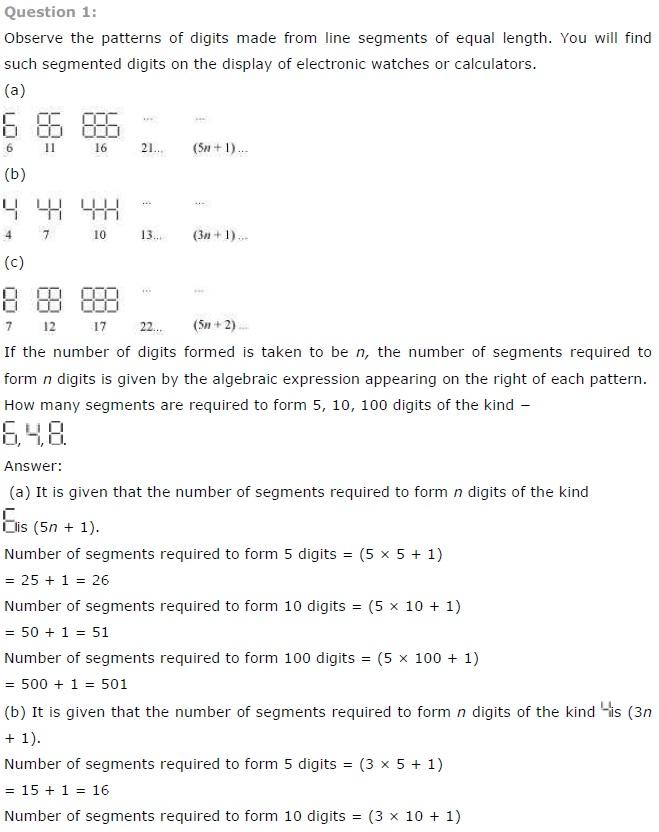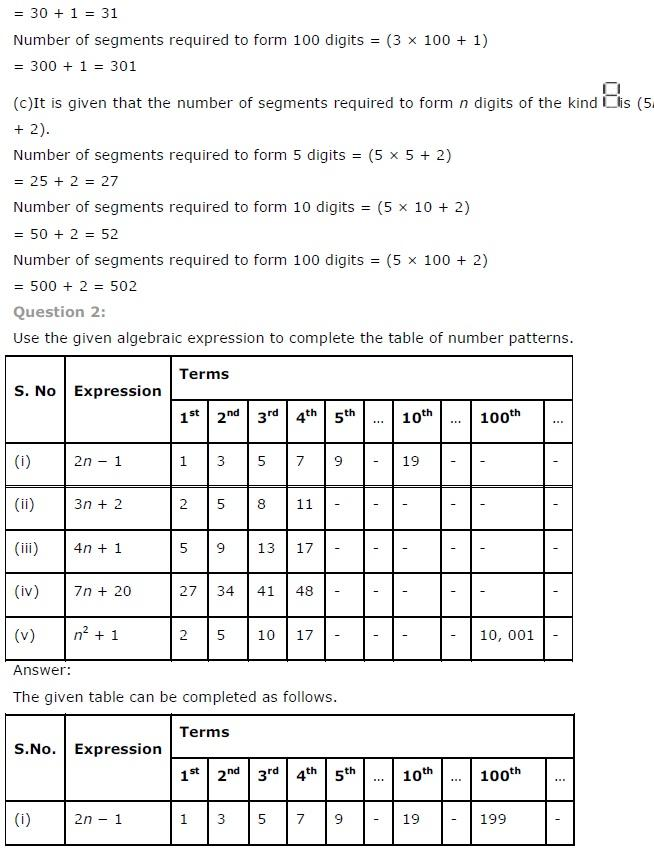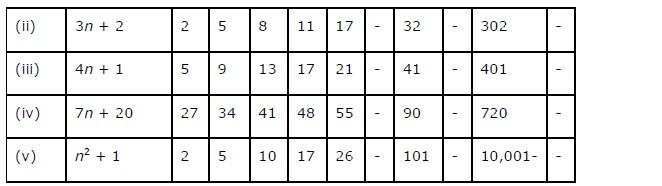# NCERT Solutions class 7 Maths Chapter-12 Exercise 12.4

## NCERT Solutions class 7 Maths Chapter-12 Algebraic expressions

### Algebraic expressions  Exercise-12.4

NCERT Solutions Class-7 Maths chapter-12 Algebraic expressions  Exercise-12.4 is prepared by academic team of pw all the questions of NCERT text book are solved step by step with proper and detail solutions explaining each and every questions . For More and additional questions of CBSE class 7 maths you can go to class 7 maths sections. NCERT class 7 Maths Solutions is the best way to enhanced your mathematics skill. And pw practice worksheet & question bank will help you a lot .

### Solutions of Chapter Algebraic expressions  Exercise-12.4NCERT CLASS 7 MATHEMATICS SOLUTIONS